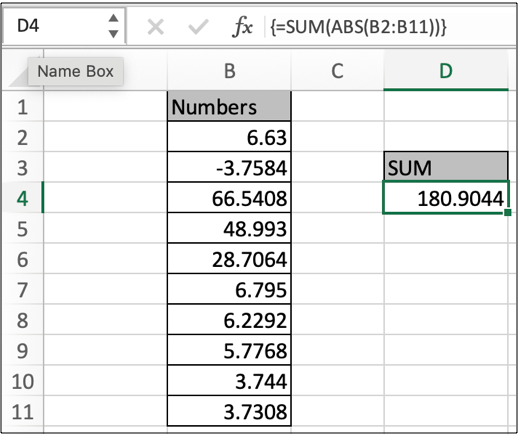# How to Sum the Absolute Values of All Numbers in a Range in ExcelIn this article, we will learn How to Sum the Absolute Values of All Numbers in a Range in Excel.

Scenario:

In Mathematics, there is an operator name absolute value. It only returns positive numbers but don't change the numerical value. For example absolute value of -12.5 be +12.5 and absolute value of +12.5 be +12.5

Excel provides a function to operate absolute value using the ABS function. For example, the square root of negative numbers is error in Excel. So use ABS function inside the square root operation in Excel. Or obtaining subtraction of two values and doesn't care about the sign of result. Let's learn ABS function syntax and an example to illustrate the function usage as shown below

Sum only positive numbers in Excel

For this we will make a formula to sum also negative values as positive values. So far sum function is an obvious choice and The ABS function just changes the negative numbers to positive numbers but doesn't change the positive number.

Formula syntax

 = { SUM(ABS(array)) }

Array : list of values given using array cell reference (A2:A502)

Note : don’t use the curly braces using the keyboard symbol. Use the Ctrl + Shift + Enter to apply the curly braces as shown below.Example :

All of these might be confusing to understand. Let's understand how to use the function using an example. Here we are given some numbers having positive and negative both distances. We just need to find the sum after having absolute of all.

Use the formula:

 { =SUM(ABS(B2:B11)) }

Note : don’t use the curly braces using the keyboard symbol. Use the Ctrl + Shift + Enter to apply the curly braces as shown below.

As you can see in the above image the sum of values is there. You can verify this. This is correct unless you have made an error.

When and how to use ABS function?

You can use this function where values are always positive. Like distance.

Supply any expression to the ABS function, which will result in a number. ABS function will return the distance of that number from 0 on the number line. And this what absolute value means

Here are all the observational notes using the formula in Excel
Notes :

1. The formula only works with numbers.
2. The formula returns the number rounded off to any decimal places.
3. Negative argument as decimal rounds the number to integer side.
4. Blank cells as an argument takes as zero value and returns 0 as result, so make sure to check blank cells before.
5. The function returns #VALUE! Error if the text value or numbers as text value.

Hope this article about How to Sum the Absolute Values of All Numbers in a Range in Excel is explanatory. Find more articles on calculating values and related Excel formulas here. If you liked our blogs, share it with your friends on Facebook. And also you can follow us on Twitter and Facebook. We would love to hear from you, do let us know how we can improve, complement or innovate our work and make it better for you. Write to us at info@exceltip.com.

Related Articles :

How to use the SUMPRODUCT function in Excel: Returns the SUM after multiplication of values in multiple arrays in excel. This function can be used to do multiple tasks. This is one of the most versatile functions.

COUNTIFS with Dynamic Criteria Range : To count with dynamic criteria range we simply use the INDIRECT function. This function can

COUNTIFS With OR For Multiple Criteria : Count cells having multiple criteria match using the OR function. To put an OR logic in COUNTIFS function you will not need to use the OR function.

Using the IF with AND / OR Functions in Microsoft Excel : These logical functions are used to carry out multiple criteria calculations. With IF the OR and AND functions are used to include or exclude matches.

How to use OR function in Microsoft Excel : The function is used to include all the TRUE values in multiple criterias.

How to Count Cells That Contain This Or That in Excel Excel :To cells that contain this or that, we can use the SUMPRODUCT function. Here's how you do those calculations.

Popular Articles :

How to use the VLOOKUP Function in Excel : This is one of the most used and popular functions of excel that is used to lookup value from different ranges and sheets.

How to use the IF Function in Excel : The IF statement in Excel checks the condition and returns a specific value if the condition is TRUE or returns another specific value if FALSE.

How to use the SUMIF Function in Excel : This is another dashboard essential function. This helps you sum up values on specific conditions.

How to use the COUNTIF Function in Excel : Count values with conditions using this amazing function. You don't need to filter your data to count specific values. Countif function is essential to prepare your dashboard.

1.